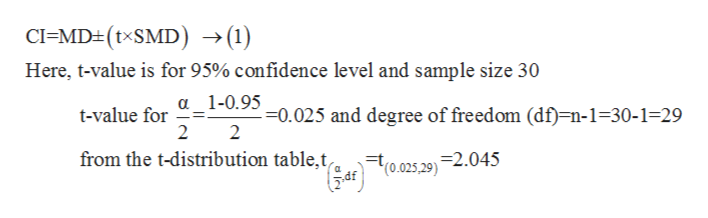# In a sample of 30 participants, a researcher estimates the 95% CI for a sample with a mean of Μ1-Μ2 = 0.7 and an estimated standard error for the difference sΜ1-Μ2 of 0.2. What is the confidence interval at this level of confidence?

Question

In a sample of 30 participants, a researcher estimates the 95% CI for a sample with a mean of Μ12 = 0.7 and an estimated standard error for the difference sΜ1-Μ2 of 0.2. What is the confidence interval at this level of confidence?

check_circleExpert Solution
Step 1

From the given question

Sample size (n) =30, mean difference (M1-M2) =0.7 and standard error difference (SM1-M2) =0.2.

Step 2

Formula for confidence interval (CI) ishelp_outlineImage TranscriptioncloseCI=MD(txSMD) >(1) Here, t-value is for 95% confidence level and sample size 30 a 1-0.95 =0.025 and degree of freedom (df)=n-1=30-1=29 2 t-value for 2 from the t-distribution table,t 2.045 (0.025,29) fullscreen
Step 3

Let substitute these values in equation...

### Want to see the full answer?

See Solution

#### Want to see this answer and more?

Solutions are written by subject experts who are available 24/7. Questions are typically answered within 1 hour*

See Solution
*Response times may vary by subject and question
Tagged in

### Statistics